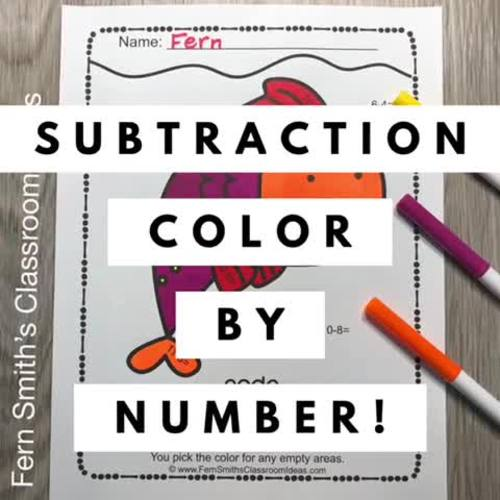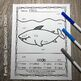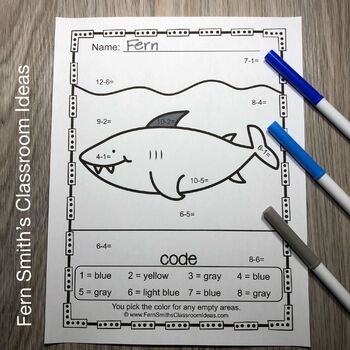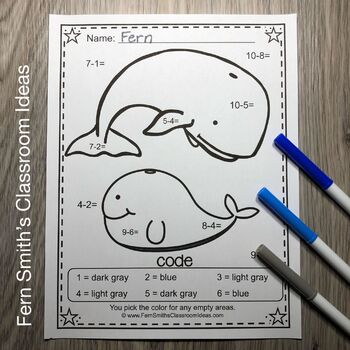digital

# Ocean Color By Number SubtractionSubject
Resource Type
Formats

TpT Digital Activity

PDF (2 MB|10 pages)
Standards
\$3.50
TpT Digital Activity
Add notes & annotations through an interactive layer and assign to students via Google Classroom.
Also included in
1. You will love the no prep, print and go ease of these Ocean Color By Number Addition, Subtraction, Multiplication, and Division Beach Fun Printables. This Ocean Color By Number Addition, Subtraction, Multiplication, and Division Printable includes 20 pages for introducing or reviewing Multiplication
\$11.20
\$14.00
Save \$2.80
2. You will love the no prep, print and go ease of these Ocean Color By Number Addition and Subtraction Beach Fun Printables. This Ocean Color By Number Addition and Subtraction Printable includes 10 pages for introducing or reviewing addition and subtraction. This bundle is perfect for differentiation
\$6.30
\$7.00
Save \$0.70
• Product Description
• Standards

You will love the no prep, print and go ease of these Ocean Color By Number Beach Fun Subtraction Printables. This Ocean Color By Number Beach Fun Subtraction Printables includes 5 pages for introducing or reviewing subtraction. Addition and Subtraction are essential skills to master in first and second grade. Students need to know how to fluently add and subtract within 20 using mental strategies as well as know from memory all sums of two one-digit numbers. Each page in this Ocean Color By Number Beach Fun Subtraction resource reinforces those skills by only focusing on two subtraction skills at a time. Your students will adore these FIVE Color By Number subtraction worksheets while learning and reviewing important subtraction skills at the same time! Each page in the resource has between 10 to 15 problems focusing on mixed subtraction skills. If you would like an Ocean Color By Number with two specific subtraction math problems on each page, click here to see my Ocean Color By Number Beach Fun Subtraction Printables. With the five Answer Keys also included, this helps you quickly and efficiently check your students work in a very short amount of time.

This Ocean Color By Number Subtraction Beach Fun Resource includes:

Five Total Pages of Student Work

1. This adorable Ocean Color By Number page has two whales swimming around in the ocean, cute, cute, cute!
2. This adorable Ocean Color By Number page has a sweet, big, old, smiling shark for your class to color!
3. This adorable Ocean Color By Number page has a lovely seahorse swimming along the ocean floor, your class will love it!
4. This cute Ocean Color By Number page has nine jellyfish and they are everywhere! Your boys and girls will love their silly smiles too!
5. This cute Ocean Color By Number page has a great big fish, with a great big smile on his great big face!

Five Matching Pages of Answer Keys Too!

*** Terrific Teaching Tip ***

Terrific for an Emergency Sub Tub or Homework!

*** CLASSROOM USE IDEAS ***

• math center assignments
• small group work
• morning work worksheet
• RTI Remediation
• seat work
• substitute teacher days
• homework
• morning work tubs
• independent practice
• early finishers
• tutoring
• clip board time worksheet
• early morning arrival time
• indoor recess

This subtraction resource is also available in these bundles:

These high interest black and white printables are great for seat work, homework, small group work, substitute teacher folders, sub tubs, the possibilities are endless!

{Please Check Out The Preview and the Cute Preview Pictures!}

A Quick Recap of What's Included for You...

Five pages of Ocean Subtraction Color By Number Beach Fun worksheets.

Five answer key pages for these Ocean Subtraction Color By Number Beach Fun worksheets.

Common Core State Standards

CCSS.MATH.CONTENT.K.OA.A.5

Fluently add and subtract within 5.

CCSS.MATH.CONTENT.1.OA.A.1

Use addition and subtraction within 20 to solve word problems involving situations of adding to, taking from, putting together, taking apart, and comparing, with unknowns in all positions, e.g., by using objects, drawings, and equations with a symbol for the unknown number to represent the problem.

CCSS.MATH.CONTENT.1.OA.B.4

Understand subtraction as an unknown-addend problem. For example, subtract 10 - 8 by finding the number that makes 10 when added to 8.

CCSS.MATH.CONTENT.1.OA.C.5

Relate counting to addition and subtraction (e.g., by counting on 2 to add 2).

CCSS.MATH.CONTENT.1.OA.C.6

Add and subtract within 20, demonstrating fluency for addition and subtraction within 10. Use strategies such as counting on, making ten, decomposing a number leading to a ten, using the relationship between addition and subtraction, and creating equivalent but easier or known sums.

CCSS.MATH.CONTENT.2.OA.B.2

Fluently add and subtract within 20 using mental strategies. By end of Grade 2, know from memory all sums of two one-digit numbers.

Click here for ALL my Color By Number / Color By Code Resources!

>>> Come join the fun on Instagram for my more FREEBIES & Monthly Giveaways.

>>> Follow my blog, Fern Smith's Classroom Ideas, where I post a Freebie Friday every Friday!

>>> Check out my Educational Pinterest Boards.

Don’t forget that all my new products are 40% off for 48 hours.

Click this LINK to follow my shop & you'll be notified of these huge savings.

Thank you,

Fern Smith *Store * Blog * Pinterest * IG * FB *

to see state-specific standards (only available in the US).
Fluently add and subtract within 20 using mental strategies. By end of Grade 2, know from memory all sums of two one-digit numbers.
Add and subtract within 20, demonstrating fluency for addition and subtraction within 10. Use strategies such as counting on; making ten (e.g., 8 + 6 = 8 + 2 + 4 = 10 + 4 = 14); decomposing a number leading to a ten (e.g., 13 - 4 = 13 - 3 - 1 = 10 - 1 = 9); using the relationship between addition and subtraction (e.g., knowing that 8 + 4 = 12, one knows 12 - 8 = 4); and creating equivalent but easier or known sums (e.g., adding 6 + 7 by creating the known equivalent 6 + 6 + 1 = 12 + 1 = 13).
Relate counting to addition and subtraction (e.g., by counting on 2 to add 2).
Understand subtraction as an unknown-addend problem. For example, subtract 10 – 8 by finding the number that makes 10 when added to 8.
Use addition and subtraction within 20 to solve word problems involving situations of adding to, taking from, putting together, taking apart, and comparing, with unknowns in all positions, e.g., by using objects, drawings, and equations with a symbol for the unknown number to represent the problem.
Total Pages
10 pages
Included
Teaching Duration
1 Week
Report this Resource to TpT
Reported resources will be reviewed by our team. Report this resource to let us know if this resource violates TpT’s content guidelines.
• Ratings & Reviews
• Q & A

Teachers Pay Teachers is an online marketplace where teachers buy and sell original educational materials.